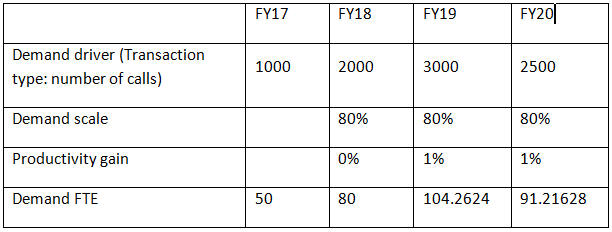## Scaling Demand FTE

If your administrator enabled the option Demand Driver Scaling Factor, you can use Demand Scale to scale your demand planning to account for a change in productivity over time. In calculating the future demand for FTE, Demand Scale factors in natural logarithm as an exponent of the ratio.

Enter a demand scale value to increase or decrease the calculated Demand FTE when using the Previous Year’s Ratio calculation logic. (Demand scale applies only if you are using Previous Year's Ratio as the calculation logic.) Demand scale enables you to increase the demand driver without increasing the resources needed using the same ratio. For example, enter 80% to reduce the calculated Demand FTE by 20% when the demand driver doubles. If you don’t enter a demand scale value, the scale is assumed to be 100% (that is, no scaling is applied).

A demand scale of n% means that for each time the demand driver doubles (for example, the number of calls to the Call Center), the productivity becomes n% of the previous year's Demand FTE, while factoring in a linear relationship of the demand scale effect.

For example, assume that FY17 is the baseline year with an FTE of 50 Call Center Operators handling 1,000 calls per year:You expect the demand driver (the number of calls to the Call Center) to double from FY17 to FY18, but the demand FTE (the number of Call Center Operators) doesn’t likewise double. In this case, you assume that a year’s experience increases the employees’ efficiency. For each doubling of the demand driver, you need only 80% of the demand FTE value because you assume a 20% gain in productivity.

The formula that Oracle Strategic Workforce Planning Cloud uses to calculate Demand FTE while factoring in Demand Scale and Expected Productivity gains is:

``((PY Total Demand FTE / PY Demand Units) * ((CY Demand Units/ PY Demand Units)^(LN(CY Demand Scale)/LN(2))) * (1 – Expected Productivity)) * CY Demand Units``

where:

• `PY` = Previous Year
• `CY` = Current Year
• `LN` = Natural Logarithm

You can further refine FTE calculations by entering values in Expected Productivity. The calculation that determines the Demand FTE factors in the values you set for both Demand Scale and Expected Productivity.